## Workshop: Introduction to Arduino

This workshop was held on October 19th 2015 for “Artificial Skins and Bones” and “Glas & Licht” semester project course.

### Installing Arduino IDE

Arduino 1.6.5
http://arduino.cc/en/Main/Software

Windows >> http://arduino.cc/en/Guide/Windows
Mac >> http://arduino.cc/en/Guide/MacOSX

After you succeed on installing the software properly, connect the Arduino with USB cable and open Blink sketch from File/Examples/Basic/BlinkThen, Upload the sketch to Arduino

Select the Arduino kind you are using from Tools/BoardSelect the the port your Arduino is connected to, from Tools/Serial Portclick the arrow button and upload the sketch to Arduino

Challenge:
Can you change the blinking speed by modifying the code?
After modifying it, upload the sketch onto the Arduino and see what happens.

Challenge:
Let’s add LED on pin 13

LED (Light Emitting Diode)
LED has direction. Be careful!
you can check the direction by looking at:
– the length of the legs. The longer one is + (Anode)
– the size of the Leadframe in the plastic casing. The smaller leadframe is + (Anode)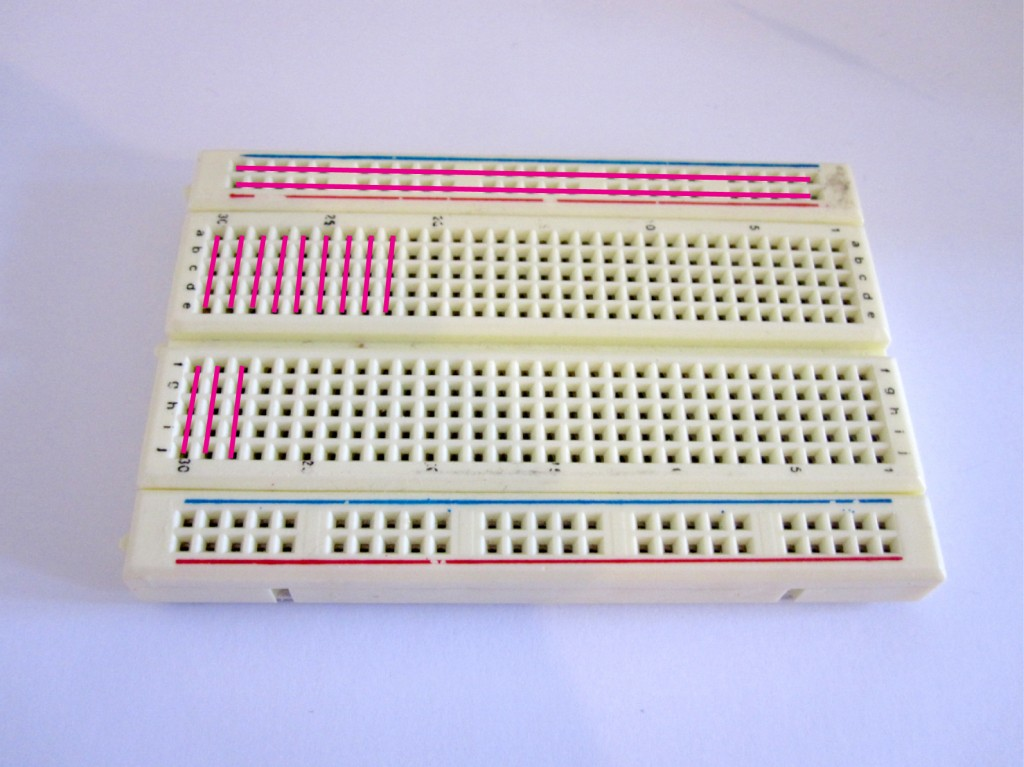Challenge:
1st LED on >> off >> on >> off
1nd LED off >> on >> off >> on

### Push Button

inside of a push buttonHow to connect push button
pull up resistor (10k ohm or bigger)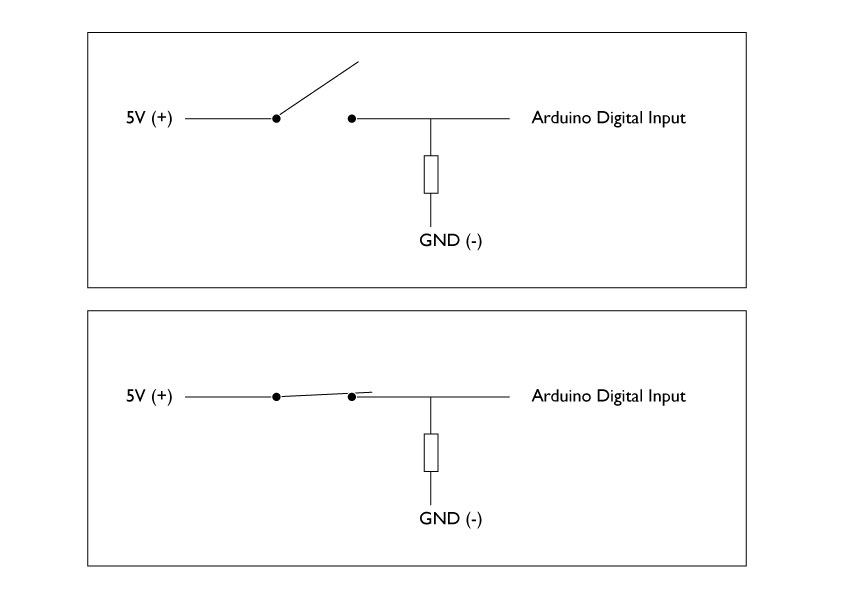Now open the Arduino Button sketch example from File/Examples/Digital/Button

In this sketch, you will see few new things
Variable
Function
if
else

Variables
A variable is a place to store a piece of data. It has a name, a value, and a type

Binary and Decimal
We use decimal system in our everyday life when dealing with numbers. Decimal is a system that uses unit of Ten or another words base as Ten. On the other hand, Binary system uses two as a base, in another words base as two.Bits and Bytes
A bit stores just a 0 or 1, and it is the smallest unit of storage
“In the computer it’s all 0’s and 1’s” … computer is “thinking” in the world of bits-binary
One byte = grouping of 8 bits

Q. What is the biggest number you can express with 1 byte? 255

Data Type
boolean (0,1) >> 1 bit
byte (0-255) >> 8 bits / 1 byte
int (-32,768 to 32,767) >> 16 bit/ 2 bytes
unsigned int (0 to 65,535) >> 16 bits/ 2 bytes
long (-2,147,483,648 to 2,147,483,647) >> 32 bits/ 4 bytes
float (as large as 3.4028235E+38 and as low as -3.4028235E+38) >> 32 bits/ 4 bytes
char ( ‘A’, ‘B’, ‘C’) >> 8 bits/ 1 byte

function
In programming, a named section of a program that performs a specific task. In this sense, a function is a type of procedure or routine. Some programming languages make a distinction between a function, which returns a value, and a procedure, which performs some operation but does not return a value.
(from http://www.webopedia.com/TERM/F/function.html)

Most programming languages come with a pre-written set of functions that are kept in a library. You can also write your own functions to perform specialized tasks.
DigitalWrite() is a pre-written function in the Arduino Library

if
if, which is used in conjunction with a comparison operator, tests whether a certain condition has been reached, such as an input being above a certain number. (from Arduino.cc)

if (the condition written here is true) then { do the thing written here }

exercise:
if ( you == student)
{ raise_your_right_hand();
if (you == female){
stand_up();
if (your_age < 25){ turn_right(); } else { turn_left(); } } else { raise_your_left_hand(); } } smile(); >> what are you doing now? are you standing? sitting? smiling?

comparison operators ==, !=, <, >
if (A == B) –if A is the same as B
if (A != B) –if A is not the same as B
if (A < B) --if A is smaller than B if (A > B) –if A is bigger than B

and you can make combinations
if (A == B && B == C) –if A is the same as B and B is the same as C
if (A == B || B == C) — if A is the same as B or B is the same as C

Let’s open an example code State Change Detection from File/Examples/Digital/StateChangeDetection
With this Sketch, the button behavior is mapped differently to the behavior of the LED than the first example.

Potentiometer
“A potentiometer, informally a pot, is a three-terminal resistor with a sliding contact that forms an adjustable voltage divider. If only two terminals are used, one end and the wiper, it acts as a variable resistor or rheostat.”
from wikipedia

inside of a potentiometer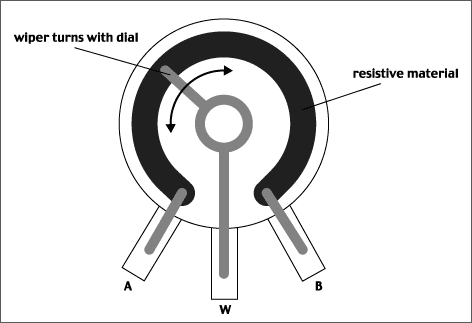Let’s connect the potentiometer to multimeter, and read out the resistance change as you turn the knob. Connect multimeter’s proves to the first pin and the middle pin of the potentiometer.

We work on this example: Analog Read Serial

introduced functions:
Serial.begin()
Serial.println()

Serial Monitorit will map input voltages between 0 and 5 volts into integer values between 0 and 1023

### Analog Write

What does it do?
Reads an analog input pin, maps the result to a range from 0 to 255 and uses the result to set the pulsewidth modulation (PWM) of an output pin.

The microcontroller’s pins can only output 0V or 5V, and not 1.2V or 2.4V. So, to output voltage between 0V-5V smoothly, in another word to do “fading” affect, it mimics the 1.2V and 2.4V by pulsing 5V and 0V quickly and change the width of the pulsation. This called PWM.

You can read the explanation at Arduino site: Pulse Width Modulation (PWM)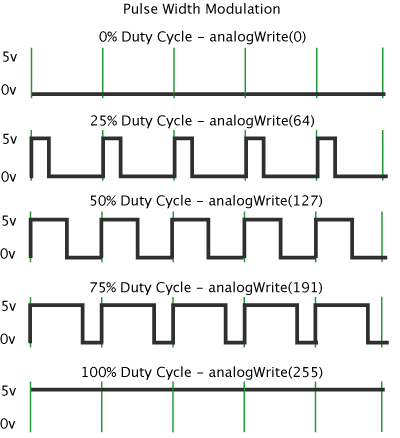Connection example: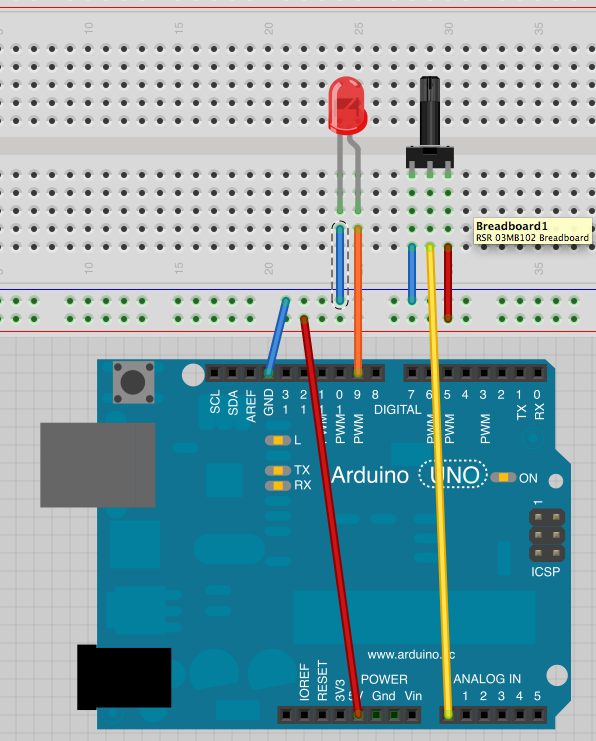introduced functions:
analogWrite()
map()